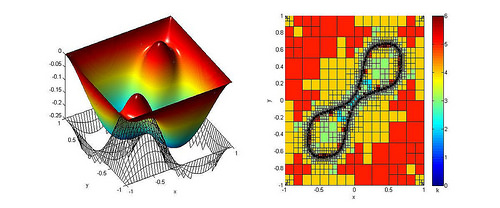## Numerial solution schemes for variational inequalitiesVariational inequalities are widely used to model technical processes as well as problems in financial mathematics. Especially contact problems, which model the deformation behaviour of contacting solids, are the focus of the studies. As variational inequalities are not resolvable explicitly, numerical discretization methods are used and implemented. Currently, the most important discretization approach for variational inequalities is based on the use of the finite-element-method (FEM), which decomposes the domain in simple geometric objects (triangles, quadrangles, tetraeder or hexaeder), as well as a polynomial approach with given continuity conditions at edges or facets. Formulations that are equivalent to variational inequalities are mixed formulations in which contact- or obstacle conditions are treated with signed Lagrange multiplier, in order to enter directly into the formulation of the variational inequality. Discretization of variational inequalities or mixed formulations lead to big systems of linear equations and inequalities, which can be transformed into non-linear equations or minimization problems. Solving this systems in an efficient way and considering the chosen discretization approach is very important when dealing with the numerical solving of variational inequalities. Solution schemes for linear equations and inequalities with more variables are already taught in school; for example, the Gaussian elimination for small systems with three to five variables. In addition, solution strategies for non-linear equations (e. g. equations with quadratic, exponential or trigonometric parts) are discussed in mathematics lessons. Higher grade students start to work with minimization problems; methods of the differential calculus help to handle these problems. Therefore, the students already familiar with the underlying problem to a certain extent. Further numerical-algorithmic aspects which will be discussed during the course of the project and were also discussed partly in maths class are interpolation problems with polynomials and the calculation of integrals with quadrature rules.
The focus of the research is the development, analysis, and the experimental evaluation of efficient numerical algorithms in order to solve variational inequalities. Basis of the research will be a mixed formulation, their solution-tuples consist of a derivative of the displacement in H(div) and a signed Lagrange multiplier in L2. This multiplier can be seen as the difference of the deformation and the obstacle function. Raviart-Thomas-finite-elements are used for the discretization of the H(div)-space, and piecewise polynomial approaches for the L2-space. Especially a finite-elements approach with polynomials of higher order is the focus of the research (p- and hp-methods). Depending on the discretization approach, the arising matrices of the resulting system of equations and inequalities show certain structural properties (for example block- or diagonal structures). The aim is to find an efficient algorithm that solves this system, considering the underlying Raviart-Thomas-discretization with finite-elements of higher order. Semismooth-Newton-methods and active-set-algorithms are especially of interest. Moreover, projective Uzawa-methods are analysed and compared with this techniques. The project’s essential innovative value is based on the development of solving-algorithms for deformation-orientated variational inequalities for the chosen gradient-orientated, mixed discretization with Riviart-Thomas-elements of higher order. The discussed topics of interest have not been implemented in the available literature yet. During the course of the project, many experiments have to be conducted in order to find the convergence characteristics and their dependence on methods- and discretization-parameters.